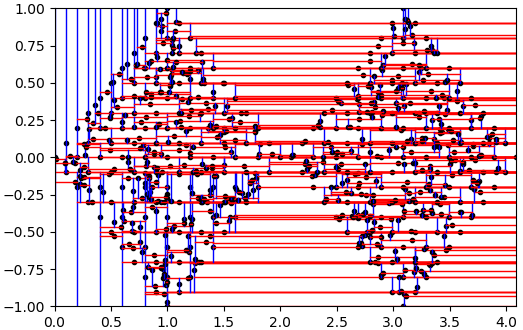pyclustering  0.10.1 pyclustring is a Python, C++ data mining library.
pyclustering.container.kdtree.kdtree_visualizer Class Reference

KD-tree visualizer that provides service to display graphical representation of the tree using matplotlib library. More...

## Public Member Functions

def __init__ (self, kdtree_instance)
Initialize KD-tree visualizer. More...

def visualize (self)
Visualize KD-tree using plot in 2-dimensional data-space.

## Detailed Description

KD-tree visualizer that provides service to display graphical representation of the tree using matplotlib library.

The visualizer is able to visualize 2D KD-trees only.

There is an example how to visualize balanced KD-tree for TwoDiamonds sample using kdtree_visualizer:

from pyclustering.container.kdtree import kdtree_balanced, kdtree_visualizer
from pyclustering.samples.definitions import FCPS_SAMPLES
tree_instance = kdtree_balanced(sample)
kdtree_visualizer(tree_instance).visualize()

Output result of the example above (balanced tree) - figure 1:Fig. 1. Balanced KD-tree for sample 'TwoDiamonds'.

There is one more example to demonstrate unbalanced KD-tree. kdtree class is child class of kdtree_balanced that allows to add points step by step and thus an unbalanced KD-tree can be built.

from pyclustering.container.kdtree import kdtree, kdtree_visualizer
from pyclustering.samples.definitions import FCPS_SAMPLES
tree_instance = kdtree() # Do not use sample in constructor to avoid building of balanced tree.
# Fill KD-tree step by step to obtain unbalanced tree.
for point in sample:
tree_instance.insert(point)
kdtree_visualizer(tree_instance).visualize()

Output result of the example above (unbalanced tree) - figure 2:Fig. 2. Unbalanced KD-tree for sample 'TwoDiamonds'.

Definition at line 19 of file kdtree.py.

## ◆ __init__()

 def pyclustering.container.kdtree.kdtree_visualizer.__init__ ( self, kdtree_instance )

Initialize KD-tree visualizer.

Parameters
 [in] kdtree_instance (kdtree): Instance of a KD-tree that should be visualized.

Definition at line 62 of file kdtree.py.

The documentation for this class was generated from the following file:
pyclustering.container.kdtree
Data Structure: KD-Tree.
Definition: kdtree.py:1
pyclustering.utils
Utils that are used by modules of pyclustering.
Definition: __init__.py:1